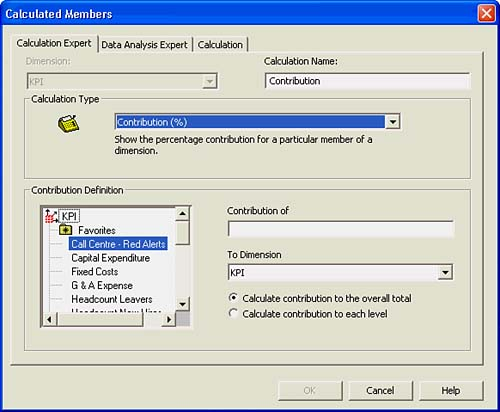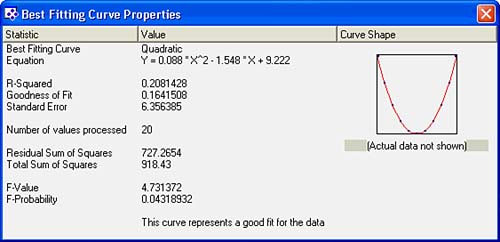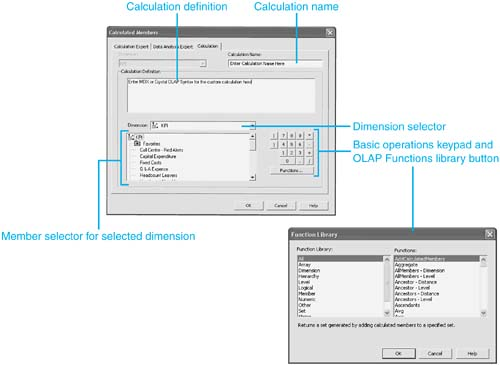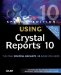# Custom Calculations and Advanced Data Analysis

Additional Calculations and Data Analytics not provided in a report's underlying data source can be added to the data presented in the worksheet through the Calculations and Data Analysis menu, which you access by right-clicking on any member header. These are available at Design time and at End-User Delivery time if context menus have been enabled on the involved Worksheet object. A number of the most common calculations are provided in the Calculated Members dialog and include drag-and-drop parameter-based experts. Additional experts are provided under the Data Analysis tab on the same Calculated Members dialog. To add any of these default calculations or to create a completely new calculation, right-click on a member or a dimension name, and then choose the Calculated Member option. This opens the Calculated Members dialog shown in Figure 20.15.

Figure 20.15. The Calculated Members dialog provides the report designer and end user with the capability to create custom calculated members not available in the OLAP data source.NOTE

Crystal Analysis automatically chooses a name for any of the predefined calculations. You can change this by typing the preferred name in the Calculation Name edit box.

The Calculation Experts

The Calculation Experts provided by Crystal Analysis on the Calculation Expert tab are

• Contribution: Calculates how much each member of a hierarchical dimension contributes to its parent. For example, how much does each week, period, and quarter contribute to total sales?
• Growth: Calculates how much a value has changed from one period to the next. For example, what is the percentage growth in sales week on week, period on period, and quarter on quarter?
• Ranking: Calculates the rank of each member in a dimension, usually based on a measure. For example, rank each product based on sales.
• Variance: Compares the value of one dimension member with a target value; the resulting variance can be expressed as an absolute value or a percentage variance.

Each of the Calculation Experts requires the specification of a different set of parameters in the Calculated Members dialog. These parameters can be set by either clicking and dragging the appropriate members to the involved parameter field or right-clicking on the chosen member and selecting the appropriate destination from the subsequent pop-up menu.

The Data Analysis Experts and Summaries

The Data Analysis Experts provided by Crystal Analysis under the Data Analysis Expert Tab are

• Trend Line: The Trend Line Expert calculates the straight line that best fits all members of the dimension specified in the Series Dimension list. This is done for the measure specified in the Trend Of box. The least squares method is used: minimizing the sum of the squares of the differences between the actual values specified and the regression line values.
• Moving Average: The Moving Average Expert calculates a centered moving average over all the members within each level of a specified dimension. This is done for the measure specified in the Moving Average parameter box.
• Linear Regression: The Linear Regression Expert calculates the straight line that best fits all the members within each level of the dimension. The members of this dimension form the columns of the Worksheet (assuming you are adding a calculated member as a row) where the X and Y values of the points are given by the members specified in the X Values box and Y Values box, respectively. The least squares method is used: minimizing the sum of the squares of the differences between the actual Y values specified and the regression line values. The regression line is evaluated at these same X values as specified by the member in the X Values box. Use the Linear Regression Expert when the data values you want to regress are not evenly spaced.

Each of the Data Analysis Experts requires you to specify a different set of parameters in the Calculated Members dialog. For a more thorough discussion on these calculations and how they are derived, please consult the Reference section of the Crystal Analysis User Manual provided in the docs directory of your install CD.

CAUTION

It is very important to understand the scope under which the Data Analysis Experts operate. When you select any of these experts, they operate across the entire set of members for the dimension that has been selected regardless of whether they are displayed on the current worksheet or viewpoint. Not taking this into account can lead to suspicious looking data when not all members are displayed. For scenarios where this assumed scope needs to be modified, the underlying MDX or Crystal OLAP Syntax created by the Data Analysis Expert can be modified under the Calculation tab of the Calculated Members dialog. This is introduced later in this chapter.

In addition to the predefined Data Analysis Experts, Crystal Analysis also provides analytic summaries such as Mean, Variance, Standard Deviation, and Best Fitting Curve. Access these summaries by right-clicking any member header and choosing Data Analysis. Figure 20.16 shows the Best Fitting Curve dialog and associated calculations.

Figure 20.16. You access supporting statistics in the Best Fitting Curve dialog box by choosing the Data Analysis menu option.For detailed insights into the statistics behind the Data Analysis summaries, review the Algorithms.pdf document distributed on the product CD.

Custom Calculations with MDX or Crystal OLAP Syntax

In addition to all the experts introduced in the previous two sections, there are times when additional calculations are required to meet a designer or end user's need. The Calculations tab highlighted in Figure 20.17 enables you to create such calculationsor, as is often the case, modify existing calculations (for example, to change the scope of application for a Moving Average or Other Calculation).

Figure 20.17. This is the Calculations tab of Calculated Members dialog and the supporting Functions Library.## Crystal OLAP Syntax and MDX

Crystal OLAP Syntax and MDX (Multi-Dimensional Expressions) are related but different syntaxes that support the definition and manipulation of multidimensional objects and data. They can be conceptually thought of as a parallel to Structured Query Language (SQL), which is used for querying relational data, but for multidimensional data sources. There is, however, no direct relationship between SQL and either MDX or Crystal OLAP Syntax. Crystal Analysis uses MDX to access SQL Server cubes and Crystal OLAP Syntax for the remaining supported data sources. Thorough descriptions can be found online for MDX at www.msdn.com (search on MDX) and in the Crystal Analysis Help file (look up Crystal OLAP in the Index tab).

Similar to an SQL query, each MDX or Crystal OLAP query requires a data request (the SELECT clause), a starting point (the FROM clause), and a filter (the WHERE clause). These and other keywords provide the tools used to extract specific portions of data from a cube for analysis. Crystal Analysis uses MDX and Crystal OLAP queries to capture data from the underlying multidimensional data sources. When using MDX (against SQL Server cubes), these queries can be viewed and edited through the Edit MDX option on the Tools menu. Additionally, both these syntaxes support extension through use of calculated members. This is generally the focal area for the report designer's exposure to MDX or Crystal OLAP and is covered through some practical examples later in this section.

The Calculations Tab consists of the four major components, shown in Figure 20.17. These components facilitate the creation of Crystal OLAP or MDX statements that can be converted into meaningful fields usable by Crystal Analysis designers and end users:

• Calculation Definition: This is the actual MDX (if you're using SQL Server) or Crystal OLAP Syntax that is calculated by or through Crystal Analysis against the underlying data source.
• Dimension and Member Selectors: These components facilitate the selection of Dimensions and Dimension Members to be used in creating the custom calculation. When dimensions or members are selected through a double-click, the appropriate syntax for referencing them is transposed into the calculation definition for future editing. It is worth noting that the transposed text might not always reflect the exact user-friendly member syntax displayed in the Member Selector.
• OLAP Functions Library: Clicking on the Functions Library button provides a library of MDX or Crystal OLAP functions that might be used in the creation of the involved custom calculation. A few of the most common and useful functions are described in the next section.
• Basic Operations Keypad: A keypad providing and basic math operations and numerics for use in creating the calculation definition.

Once created, a resultant MDX or Crystal OLAP definition created through the Calculations tab appears as just another member in the involved Crystal Analysis report--which could even be used in future custom calculations.Special Edition Using Crystal Reports 10
ISBN: 0789731134
EAN: 2147483647
Year: 2003
Pages: 341